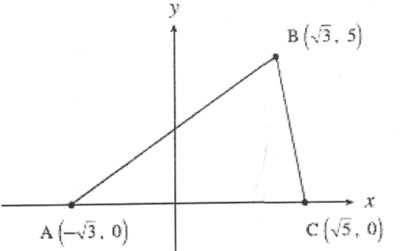SEARCH HOMEMath Central Quandaries & QueriesHi, I have attached figure of the triangle, Can you please help to find the area of Triangle ABC. Thanks. AshiyaHi Ashiya.Remember that the area of any triangle can be found if you know the base length and the height. In this case, it is easy to see the height, because point B has a y value of 5 and the points on the base have a y value of 0, so the height is just 5-0 = 5 units.

The base length is the length of the side AC. Can you see how to find it? The square roots might make things look confusing, but what would you do if they weren't there [what if A was at (-3,0) and C was at (5,0)? Wouldn't you just subtract: 5 - (-3) = 8 units?] - you can do the same thing, but follow the rules for working with square roots.

When you determine the base length, just remember that the area of a triangle is 1/2 x base length x height.

Hope this helps,
Stephen La RocqueMath Central is supported by the University of Regina and The Pacific Institute for the Mathematical Sciences.